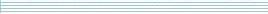| home      | people     | research     | publications    | seminars    | events     | contactCommunication Networks   | Systems Biology   | Hybrid Systems    | Machine Learning   | Dynamics & Interaction### Schools Mathematics Grand Challenge

Week six's Puzzles

Problem 16:

The sixteenth problem was:

One Saturday morning, Mary cycled from her home in Dublin to Maynooth, and then cycled home along the same route that afternoon. The distance from her home to Maynooth is exactly 10 miles and she cycled at a constant speed of 10 miles per hour in both directions. On Sunday, she decided to make the same cycling trip. However, due to a westerly wind, she cycled at a constant speed of 5 miles per hour when going from Dublin to Maynooth, and at a speed of 15 miles per hour on the return trip from Maynooth to Dublin.

How much longer, in minutes, did the trip take on Sunday?

The solution was:

The answer is that the Sunday trip took 40 minutes longer than usual.

Let be x the time needed for the full trip under normal conditions. Then since the speed is constant (10 miles per hour), the total trip takes

x = (20miles)/(10miles/hour) = 2 hours.

Now, let y be the time needed for the Sunday trip. Since the speed from Dublin to Maynooth was 5 miles per hour, and the speed from Maynooth to Dublin going east was 15 miles per hour we have that the Sunday trip took

y = (10miles)/(5miles/hour) + (10miles)/(15miles/hour) = 2hours + 2/3hour.

Thus, the Sunday trip took y-x = 2/3hours = 40 minutes longer than usual.

Problem 17:

The seventeenth problem was:

The following week, Mary cycled off in another direction on Saturday morning. She left her home at 9 am and cycled 60 miles at a constant speed of 7 miles per hour until she reached a guesthouse. She spent Saturday night in the guesthouse and cycled back home on Sunday morning, starting from the guesthouse at 9 am and cycling back home along the same route as the day before at a constant speed of 13 miles per hour.

The solution of a well-known puzzle says that Mary will pass some point along the route at exactly the same time on both Saturday and Sunday. How many hours after 9 am will this happen?

TIP: When you've figured out the time, remember to check that Mary will be the same distance from home at that time on both days.

The solution was:

The solution was 3 hours.

Let z be the amount of time after 9 am, when Mary was at the same point both on Saturday and Sunday. We know that

z*7miles/hour+z*13miles/hour = 60 miles.

Thus, z*20/hour = 60, so z = 3 hours.

Problem 18:

The eighteenth problem was:

In this problem, the notation A / B means A divided by B.

Jim recently bought a rather odd computer game, which works as follows. First of all, Jim picks a positive whole number A. The computer then must pick a positive whole number B which is strictly less than A (0 < B < A) and so:

(A / B) + (30 / A) is the same as (B / A) + (30 / B).

If it is possible to pick such a number, the computer will do so and the computer then wins. Otherwise, if the computer cannot find any such whole number B, Jim wins the game.

Jim played the game 20 times, using all the whole numbers between 1 and 20 inclusive. The first time, he chose the number 1, the second time the number 2, and so on up to the twentieth time, when he chose 20. How many times did Jim win?

The solution was:

The solution was: Jim won 15 times.

If Jim picks A, the computer wins if and only if there is a whole number B greater than 0 and less than A such that:

A/B + 30/A = B/A + 30/B.

The previous equality is satisfied if and only if

(A*A + 30B)/AB = (B*B + 30A)/AB

which holds if and only if

A*A -B*B = 30(A-B).

The last is equivalent to

(A-B)*(A+B) = 30(A-B).

Since B must be strictly less than A, we know that A-B>0 and therefore the computer wins if and only if there is a whole number B greater than 0 and less than A such that:

A+B = 30.

For A = 1,2,3,4,5,6,7,8,9,10,11,12,13,14,15 there is no B less than A such that A+B = 30 and in these 15 cases Jim wins. However, for A = 16,17,18,19,20 the computer takes B = 30-A which is strictly greater than zero and less than A, and therefore Jim loses in these five games.